# Convex body chasing, Steiner point, Sellke point, and SODA 2020 best papers

Big congratulations to my former intern Mark Sellke, and to the CMU team (C. J. Argue, Anupam Gupta, Guru Guruganesh, and Ziye Tang) for jointly winning the best paper award at SODA 2020 (as well as the best student paper for Mark)! They obtain a linear in the dimension competitive ratio for convex body chasing, a problem which was entirely open for anyjust two years ago. What’s more is that they found the algorithm (and proof) from The Book! Let me explain.

Convex body chasing

In convex body chasing an online algorithm is presented at each time step with a convex body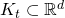, and it must choose a point. The online algorithm is trying to minimize its total movement, namely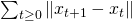(all the norms here will be Euclidean, for simplicity). To evaluate the performance of the algorithm, one benchmarks it against the smallest movement one could achieve for this sequence of bodies, if one knew in advance the whole sequence. A good example to have in mind is if the sequence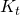corresponds to lines rotating around some fixed point, then the best thing to do is to move to this fixed point and never move again, while a greedy algorithm might have infinite movement (in the continuous time limit). The competitive ratio is the worst case (over all possible sequences of convex bodies) ratio of the algorithm’s performance to those of the oracle optimum. This problem was introduced in 1993 by Linial and Friedman, mainly motivated by considering a more geometric version of the-server problem.

As it turns out, this problem in fact has a lot of “real” applications. It is not too surprising given how elementary the problem is. Just to give a flavor, Adam Wierman and friends show that dynamic powering of data centers can be viewed as an instance of convex *function* chasing. In convex function chasing, instead of a convex body one gets a convex function, and there is then a movement cost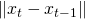and a *service cost*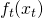. While this is more general than convex body chasing, it turns out that convex body chasing in dimension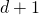is enough to solve convex function chasing in dimension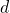(this is left to the reader as an exercise). Roughly speaking in dynamic power management, one controls various power levels (number of servers online, etc), and a request correspond to a new set of jobs. Turning on/off servers has an associated cost (the movement cost), while servicing the jobs with a certain number of servers has an associated delay (the service cost). You get the idea.

The Steiner point

In a paper to appear at SODA too, we propose (with Yin Tat Lee, Yuanzhi Li, Bo’az Klartag, and Mark Sellke) to use the Steiner point for the nested convex body chasing problem. In nested convex body chasing the sequence of bodies is nested, and to account for irrelevant additive constant we assume that the oracle optimum starts from the worst point in the starting set. In other words, opt is starting at the point the furthest away infrom the closest point in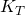, so its movement is exactly equal to the Hausdorff distance betweenand. So all we have to do is to give an online algorithm whose movement is proportional to this Hausdorff distance. Some kind of Lipschitzness in Hausdorff distance. Well, it turns out that mathematicians have been looking at this for centuries already, and Steiner back in the 1800’s introduced just what we need: a selector (a map from convex sets to points in them, i.e.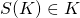for any convex set) which is Lipschitz with respect to the Hausdorff distance, that is: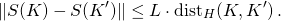Note that obtaining such a selector is not trivial, for example the first natural guess would be the center of gravity, but it turns out that this is not Lipschitz (consider for example what happens withbeing a very thin triangle and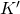being the base of this triangle). Crazy thing is, this Steiner point selector (to be defined shortly) is not only Lipschitz, it is in fact the most Lipschitz selector for convex sets. How would you prove such a thing? Well, you clearly need a miracle to happen, and the miracle here is that the Steiner point satisfies some symmetries, which define it uniquely. From there all you need to do is that starting with any selector, you can add some of these symmetries while also improving the Lipschitz constant (for more on this see the references in the linked paper above).

OK, so what is the Steiner point? It has many definitions, but a particular appealing one from an optimizer’s viewpoint is as follows. For a convex setand a vectorlet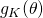be the maximizer of the linear function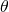in the set. Then the Steiner point of,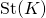is defined by: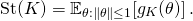In words: for any direction take the furthest point inin that direction, and average over all directions. This feels like a really nice object, and clearly it satisfies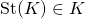(i.e., it is a selector). The algorithm we proposed in our SODA paper is simply to move to. Let us now see how to analyze this algorithm.

The nested case proof

First we give an alternative formula for the Steiner point. Denote, and observe that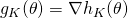for almost all. Thus using the divergence theorem one obtains:(The factorcomes from the ratio of the volume of the ball to the sphere, and thein the expectation is because the normal toison the sphere.)

Now we have the following one-line inequality to control the movement of the Steiner point: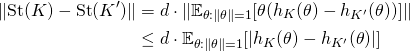It only remains to observe thatto obtain a proof that Steiner is-Lipschitz (in fact as an exercise you can try to improve the above argument to obtain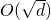-Lipschitz). Now for nested convex body chasing we will have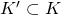so that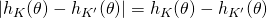(i.e., no absolute values), and thus the upper bound on the movement will telescope! More precisely:This proves that the Steiner point is-competitive for nested convex body chasing. How to generalize this to the non-nested case seemed difficult, and the breakthrough of Mark and the CMU team is to bring back an old friend of the online algorithm community: the work function.

The work function

The work function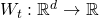is defined as follows. For any pointit is the smallest cost one can pay to satisfy all the requests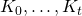and end up at. First observe that this function is convex. Indeed take two pointand, andthe middle point betweenand(any point on the segment betweenandwould be treated similarly). In the definition of the work function there is an associated trajectory for bothand. By convexity of the requests, the sequence of mid points between those two trajectories is a valid trajectory for! And moreover the movement of this mid trajectory is (by triangle inequality) less than the average of the movement of the trajectory forand. Hence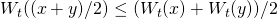.

A very simple property we will need from the work function is that the norm of its gradient carries some information on the current request. Namely,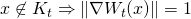(indeed, ifis not in the current request set, then the best way to end up there is to move to a pointinand then move to, and ifis a polytope then when you move a little bityou don’t move, so the cost is changing at rate, hence the norm of the gradient being). Or to put it differently: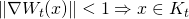.

The Sellke point

Mark’s beautiful idea (and the CMU team very much related –in fact equivalent– idea) to generalize the Steiner point to the non-nested case is to use the work function as a surrogate for the request. Steiner ofwill clearly not work since all ofdoes not matter in the same way, as some points might be essentially irrelevant because they are very far from the previous requests, a fact which will be uncovered by the work function (while on the other hand the random direction from the Steiner point definition is oblivious to the geometry of the previous requests). So how to pick an appropriately random point inwhile respecting the work function structure? Well we just saw that. So how about taking a random direction, and applying the inverse of the gradient map, namely the gradient of the Fenchel dual? Recall that the Fenchel dual is defined by:This is exactly the algorithm Mark proposes and it goes like this (Mark calls it the *functional Steiner point*):Crucially this is a valid point, namely. Moreover just like for the Steiner point we can apply the divergence theorem and obtain:The beautiful thing is that we can now exactly repeat the nested convex body argument, since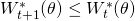for all(just like we had in the nested case) and so we get:The first term with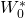is just some additive constant which we can ignore, while the second term is bounded as follows:Thus we exactly proved that the movement of the Sellke point is upped bounded by a constant plustimes the minimum of the work function, and the latter is nothing but the value of the oracle optimum!

Note that once everything is done and said, the proof has only two inequalities (triangle inequality as in the nested case, and the minimum of the expectation is less than the expectation of the minimum). It doesn’t get any better than this!

This entry was posted in Theoretical Computer Science. Bookmark the permalink.

## 3 Responses to "Convex body chasing, Steiner point, Sellke point, and SODA 2020 best papers"

•••